Queries for Skew partitions: search statistic / browse statistics / browse maps from / browse maps to

# 1. Definition & Example

• A skew partition $(\lambda,\mu)$ of $n \in \mathbb{N}_+$ is a pair of integer partitions such that $\mu \subseteq \lambda$ as Ferrers diagrams.

• Skew partitions are graphically represented by their Ferrers diagram (or Young diagram) as the collection of boxes of $\lambda$ that are not boxes of $\mu$.

• A skew partition is reduced if its Ferrers diagram does not contain empty rows before the last nonempty row and empty columns before the last nonempty column.

• We write $(\lambda,\mu) \vdash n$ if $\lambda$ is a partition of $n$.

 the 9 Skew partitions of size 3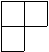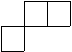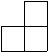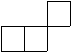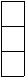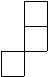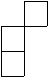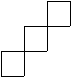[,[]] [[2,1],[]] [[3,1],] [[2,2],] [[3,2],] [[1,1,1],[]] [[2,2,1],[1,1]] [[2,1,1],] [[3,2,1],[2,1]]
• The number of skew partitions is A225114.

TBA

# 5. Technical information for database usage

• A skew partition is uniquely represented as a list of pairs representing the two integer partitions.

• Skew partitions are graded by the size of the bigger partition minus the size of the smaller one.
• The database contains all integer partitions of size at most 7.